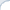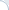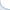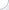## Efficient exponentiation by square rooting

Recently, I was discussing the problem of efficient exponentiation to integer powers. As I learned, the GNU library (apparently aiming for maximum precision) cannot carry out this operation efficiently. One can do better by doing exponentiation by squaring. What if the power is not integer, but fractional? The GNU library is working super slowly in this case and I cannot get more than miserly 20 million of exponentiations per second even on a modern Core i7.

What if I do not care about exponentiating efficiently for all the powers, but only for some rational ones? Can I do better? For example, I want to play with similarity search in pesky non-metric Lp spaces (for p < 1) and I can select only convenient values of p such as 1/2, 1/4, or 1/8. If I multiply these values by 8, I obtain integer numbers. One can see, and it is sure a very old idea, that we can use exponentiation by square rooting here. For instance, to compute the power 1/8, we can apply the square root three times. For the power 1/2 + 1/4, we need to obtain the square root and memorize it. Then, we apply the square root two more times and multiply the result by the already computed square root.

This algorithm relies on the binary representation of the exponent and works for the same reason as exponentiation by squaring. But this should be a crazy idea, because computing a square root is a costly operation, right? No, it is probably not. In many cases, square rooting takes about 2-3 CPU cycles and can be two orders of magnitude faster than the function pow!

I wrote a simple test to verify my hypothesis. As usual, I compile using both GCC and Intel. To prevent Intel and GNU from "cheating", I sum up all computed distances and print the result in the end. This is a good-enough trick for the GNU compiler, but not for the Intel compiler. If the variable sum becomes very large, the Intel-generated code is smart enough to stop computing powers, which defeats the purpose of testing. This is why I additionally adjust the variable sum through multiplying it by small constants. Adjustment operations do introduce little overhead, but it is very small compared to the cost of exponentiation. And, of course, we are careful enough not to use division:

    for (int j = 0; j < rep; ++j) {        for (int i = 0; i < N*4; i+=4) {            sum += 0.01 * pow(data1[i],   data2[i]);            sum += 0.01 * pow(data1[i+1], data2[i+1]);            sum += 0.01 * pow(data1[i+2], data2[i+2]);            sum += 0.01 * pow(data1[i+3], data2[i+3]);        }        sum *= fract;    }

Some benchmarking highlights:

1. In my test, exponentiation by squaring either matches performance of the GNU pow or is substantially faster;
2. When I plug the improved pow into the actual code for nearest neighbor search in pesky Lp (p<1) spaces, it provides more than a five-fold speed-up.
3. Exponentiation by squaring can even be faster than the Intel's function pow for exponent with less than 5 binary digits (if you compile the code using the Intel's compiler).

Notes on precision. This version produces results, which are almost identical to the results from the GNU function. If we can tolerate an approximate version (with a relative error about 10-5, there are more efficient solutions).

## Is Java memory efficient?

This was inspired by a real task of sorting 25 million URLs. The topic of memory efficiency in Java is old. Yet, running out of memory and making the garbage collector to consume 700% of CPU (in a futile attempt to find free memory) is never old. So, I decided to run my own tests: Can I sort 10 and 50 million URLs in the memory of my computer?

Test setup: 16 GB of memory, Intel Core i7 laptop CPU, 3.3 Ghz peak frequency. I use Oracle JVM 1.7, but similar results are obtained using OpenJDK 1.7. The maximum memory size for the JVM is set to 16 GB (through JVM flags). Java is built and run using Maven. Maven displays memory usage. In addition, the peak memory usage of both C++ and the Java program is obtained through a custom utility, which checks memory consumption 10 times per second. The code is available online.

Each URL is about 50 characters. I generate strings whose lengths are random and uniform numbers from 40 to 60. Then, I create an array where I save references to two-element objects. One element is a URL (String type). Another element is an ID (int). I am careful enough to create each string only once. Overall, there are two test cases with 10 and 50 million strings, respectively.

In addition, I wrote a C++ program that does the same thing: randomly generates objects containing short strings (URLs) and integer ids. Then it saves object references in a fixed-size array (more precisely, you use pointers in C++). In Java a character uses 16 bits (two bytes). Thus, in the C++ program I allocate strings that are twice as long as that in the Java program. I also explicitly delete all the memory before exiting the C++ program.

For 10 million strings, the data needs 0.96 GB to be stored (without overhead). The other statistics is:

 Peak memory usage (Gbs) Run-time (secs) Java 3 18.4 C++ 1.68 2.7

For 50 million strings, the data needs about 5 GB to be stored (without overhead). The other statistics is:

 Peak memory usage (Gbs) Run-time (secs) Java 10.5 120 C++ 8.42 11

As you can see, in this simple example, Java is a somewhat "greedier" than C++, but not terribly so. When the number of strings is smaller (10 million), the Java program uses thrice as many bytes as it needs to store data (without overhead). For the larger data set, the Java program the overhead coefficient is only 2.1. For C++, the overhead coefficient is roughly 1.7 in both cases. As advised by one of my readers, even smaller footprint in Java can be achieved by using specialized libraries. One good example is the FastUtil library. What such libraries typically do: they pack many strings/objects tightly in a byte array.

Note that the run-time of the Java program is considerable. As advised in the comments, it takes much shorter time to run, if we increase the amount of memory initially allocated by the garbage collector (option -Xms). However, in this case, it is harder to measure the amount of memory consumed by a Java program.

## Is integer division faster than floating-point division?

I discussed my previous post with Daniel Lemire and he pointed out that integer division was also very slow. This really surprised me. What is even more surprising is that there is no vectorized integer division. Apparently, not in even in AVX2. As noted by Nathan Kurz, integer division is so bad that you can probably do better by converting integers to floating-point numbers, carrying out a vectorized floating-point division operation, and casting the result back to integer.

So, I decided to verify this hypothesis. Unfortunately, it is not possible to use single-precision floating point numbers for all possible integer values, because the significand can hold only 23 bits. This is why my first implementation uses double-precision values. Note that I implemented two versions here: one uses 128-bit vector operations (SSE4.1) and another uses 256-bit vector operations (AVX). The code is available online. The double-precision test includes functions: testDiv32Scalar, testDiv32VectorDouble, and testDiv32VectorAVXDouble. The results on (my laptop Core i7) are:

testDiv32Scalar

Milllions of 32-bit integer DIVs per sec: 322.77

testDiv32VectorDouble

Milllions of integer DIVs per sec: 466.964

testDiv32VectorAVXDouble

Milllions of integer DIVs per sec: 374.595

As you can see, there is some benefit of using SSE extensions, but not AVX. This is quite surprising as many studies found AVX to be superior. Perhaps, this is due to the fact that AVX load/stores are costly and AVX cannot outperform SSE unless the number of load/store operations is small compared to to the number of arithmetic operations.

If we don't need to deal with numbers larger than 222, single-precision format can be used. I implemented this idea and compared the solution based on division of single-precision floating-point numbers against division of 16-bit integer numbers. We are getting a three-fold improvement with SSE and only a two-fold improvement with AVX:

testDiv16Scalar

Milllions of 16-bit integer DIVs per sec: 325.443

testDiv16VectorFloat

Milllions of 16-bit integer DIVs per sec: 997.852

testDiv16VectorFloatAvx

Milllions of 16-bit integer DIVs per sec: 721.663

It is also possible to do divide integers using several CPU instructions. This approach relies on clever math, but can it be faster than a built-in CPU operation? Indeed, it can, if one computes several quotients at once using SSE/AVX instructions. This method is implemented in the Intel math library (function _mm_div_epi32) and in the Agner's library vectorclass. In the latter, all vector elements can be divided only by the same divisor. The Intel library allows you to specify a separate divisor for each vector element. On core i7, the Agner's function is only 10% faster than built-in scalar division. The Intel's function is about 1.5 times faster than scalar division. Yet, it is about 1.5 times slower than the version based on single-precision numbers.

Finally, I carried out some tets for an AMD CPU and observed higher performance gains for all the methods discussed here. In particular, the version that relies on double-precision numbers is 4 times faster than the scalar version. The Agner's vectorclass division is twice is fast as the scalar version.

## Is division slower than multiplication?

I was discussing efficiency issues, related to nearest-neighbor searching, with Yury Malkov. One topic was: "is division slower than multiplication?" I personally believed that there should not be much difference, as in the case of other simple arithmetic operations. Yury pointed out that division was much slower. In particular, according to of Agner Fog, not only division has high latency (~20 CPU cycles), but also a low throughput (0.05 division per CPU cycle).

As I explained during my presentation at SISAP 2013, optimizing computation of a distance function can be much more important than designing a new data structure. In that, efficient computation of quotients can be crucial to some distance functions. So, it is important to know if multiplication is faster than division. If it is faster, then how much faster?
This topic is not new, but I have not found sufficiently thorough tests for recent CPUs. Thus, I have run my own and have come to a conclusion that multiplication is, indeed, faster. Division has higher latency than multiplication and in some cases this difference can be crucial. In my tests, there was a 2-6x difference. Last, but not least, when there are data dependencies, multiplication can also be slow and take several CPU cycles to complete. The details are below.

The code can be found on GitHub (module testdiv.cpp). To compile, I used the following command (the flag -Ofast is employed):

 make -f Makefile.gcc_Ofast

Even though there is a makefile for the Intel compiler, I don't recommend using it. Intel can "cheat" and skip computations. I tested using the laptop version of Core i7. Similar results were obtained using the server version of Core i7 and a relatively modern AMD processor. Note that, in addition, to complete runtime, I compute the number of divisions/multiplications per second. This is somewhat inaccurate, because other operations (such as additions) also take some time. Yet, because multiplications and divisions appear to be considerably more expensive than other operations, this can serve as a rough indicator of throughput in different setups.

In the first test (testDivDataDep0), I deliberately introduce data dependencies. The result of one division becomes an argument of the next one:

 for(size_t i = 0; i < rep; ++i) {     c1+=b1/c4;     c2+=b2/c1;     c3+=b3/c2;     c4+=b4/c3; }

Similarly, see the function testMulDataDep0, I introduce dependencies for the multiplication:

for(size_t i = 0; i < rep; ++i) {    c1+=b1*c4;    c2+=b2*c1;    c3+=b3*c2;    c4+=b4*c3;}

The functions testMulMalkovDataDep0 and testDivMalkovDataDep0 are almost identical, except one uses multiplication and another uses division. Measurements, show that testDivMalkovDataDep, which involves division, takes twice as long to finish. I can compute about 200 million divisions per second and about 400 million multiplications per second.

Let's now rewrite the code a bit, so our dependencies are "milder": To compute an i-th operation, we need to know the result of the (i-4)-th operation .The function to test efficiency of division (testDivDataDep1) now contains the following code:

for(size_t i = 0; i < rep; ++i) {    c1+=b1/c1;    c2+=b2/c2;    c3+=b3/c3;    c4+=b4/c4;}

Similarly, we modify the function to carry out multiplications (testMulDataDep1):

for(size_t i = 0; i < rep; ++i) {    c1+=b1*c1;    c2+=b2*c2;    c3+=b3*c3;    c4+=b4*c4;}

I can now carry out 450 million divisions and 2.5 billion multiplications per second. The overall runtime of the function that tests efficiency of division is 5 times as long compared to the function that tests efficiency of multiplications. In addition, the run-time of the function testMulMalkovDataDep0 is 5 times as long as the runtime of the function testMulMalkovDataDep1. To my understanding, the reason for such a difference is that computation of multiplications in testMulMalkovDataDep0 takes much longer than in testMulMalkovDataDep1 (due to data dependencies). What can we conclude at this point? Apparently, the latency of division is higher than that of multiplication. However, in the presence of data dependencies, multiplication can also be slow and take several CPU cycles to complete.

To conclude, I re-iterate that there appears to be some difference between multiplication and divisions. This difference does exist even in top-notch CPUs. Further critical comments and suggestions are appreciated.

Acknowledgements: I thank Yury Malkov for the discussion and scrutinizing some of my tests.

UPDATE 1: I have also tried to implement an old trick, where you reduce the number of divisions at the expense of carrying out additional multiplications. It is implemented in the function testDiv2Once. It does help to improve speed, but not always. In particular, it is not especially useful for single-precision floating-point numbers. Yet, you can get a 60% reduction of run-time for the type long double. To see this, change the value of constant USE_ONLY_FLOAT to false.

UPDATE 2: It is also interesting that vectorization (at least for single-precision floating-point operations) does not help to improve the speed of multiplication. Yet, it may boost the throughput of division in 4 times. Please, see the code here.

UPDATE 3: Maxim Zakharov ran my code for the ARM CPU. And the results (see the comments) are similar to that of Intel: division is about 3-6 times slower than multiplication.

## Is statistical significance useful?

There is an opinion that a statistical test is merely a heuristic with good theoretical guarantees. In particular, because, if you take a large enough sample, you are likely to get a statistically significant result. Why? For instance, in the context of information retrieval systems, no two different systems have absolutely identical values of the mean average precision or ERR. A large enough sample would allow us to detect this situation. If a large sample can get us a statistically significant result, is statistical testing useful?

First of all, in the case of one sided tests, adding more data may not lead to statistically significant results. Imagine, that a retrieval system A is better than a retrieval system B. We may have some prior beliefs that B is better than A and, therefore, we try to reject the hypothesis that B is worse than A. Due to high variance in query-specific performance scores, it may be possible to reject this hypothesis for a small set of queries. However, if we take a large enough sample, such rejection would be unlikely.

Let us now consider two-sided tests. In this case, you are likely to "enforce" statistical significance by adding more data. In other words, if systems A and B have slightly different average performance scores, we will able to select a large enough sample of queries to reject the hypothesis that A is the same as B. However, because the sample is large, the difference in average performance scores will be measured very reliably (most of the time). Thus, we will see that the difference between A and B is not substantial. In contrast, if we select a small sample, we may accidentally see a large difference between A and B, but this difference will not be statistically significant.

So, what is the bottom line? Statistical significance may be a heuristic, but, nevertheless, a very important one. If we see a large difference between A and B that is not statistically significant, then the true difference between in average performance between A and B may not be substantial. The large difference observed for a small sample of queries can be due to a high variance in query-specific performance scores. And, if we measure average performance between A and B using a large sample of queries, we may be able to detect a statistically significant difference, but the difference in performance will not be substantial. Or, alternatively, we can save the effort (evaluation can be very costly!) and do something more useful. This would be a benefit of carrying out a statistical test (using a smaller sample).

PS: Another concern related to statistical significance testing is "fishing" for p-values. If you do multiple experiments, you can get a statistically significant result by chance. Sometimes, people just discard all failed experiments and stick with a few tests where, e.g., p-values < 0.05. Ideally, this should not happen: One needs to adjust p-values so that all experiments (in a series of other relevant tests) are taken into account. Some of the adjustments methods are discussed in the previous blog post.

## Pages